Next: Initial Conditions on the Up: Scale Factor Evolution Previous: Correcting for Staggered Leapfrog

## Power-Law Expansion

LATTICEEASY is designed to self-consistently solve for the evolution of scalar fields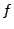and the scale factor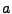in an expanding universe. In some cases, however, you may wish to solve for the behavior of a set of fields in a universe dominated by other forms of energy, e.g. pure matter or radiation. In this case you can tell the program to impose a fixed power-law expansion and evolve the fields in this background. In this section we derive the equations for such an expansion in program variables. Note that we use the variablesto denote constants of the equations. Thein this section have no relation to the ones in the previous (or any other) section.

For a general constant equation of state the scale factor evolution is given by(6.37)

The program time is rescaled as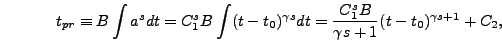(6.38)

which can be inverted to give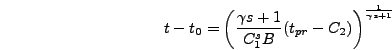(6.39)

and thus(6.40)

To solve for the parameterswe want to match the values ofandat the beginning of the simulation,. The scale factor itself has an arbitrary scaling and is set to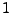initially, while the Hubble constant has some well defined initial value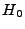. The first constraint trivially gives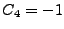. The second constraint is most easily defined in terms of the program value of the Hubble constant,(6.41)

Let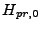be the value of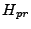when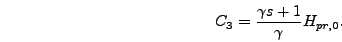(6.42)

So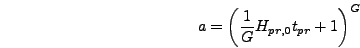(6.43)

where(6.44)

The program valueis derived in section 6.3.6 and is automatically calculated by the program. The rescaling variable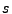should be defined for your model, so all you need for a power-law expansion is to specify the value of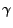, which is declared in parameters.h with the variable name expansion_power. Note that if you know the equation of state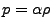that you want the corresponding power-law expansion will be given by(6.45)

(See for example .) If we let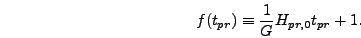(6.46)

then the final form of the power-law expansion equations is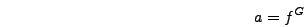(6.47)(6.48)(6.49)

The parametersand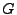are called sfbase and sfexponent respectively in the program.Next: Initial Conditions on the Up: Scale Factor Evolution Previous: Correcting for Staggered Leapfrog